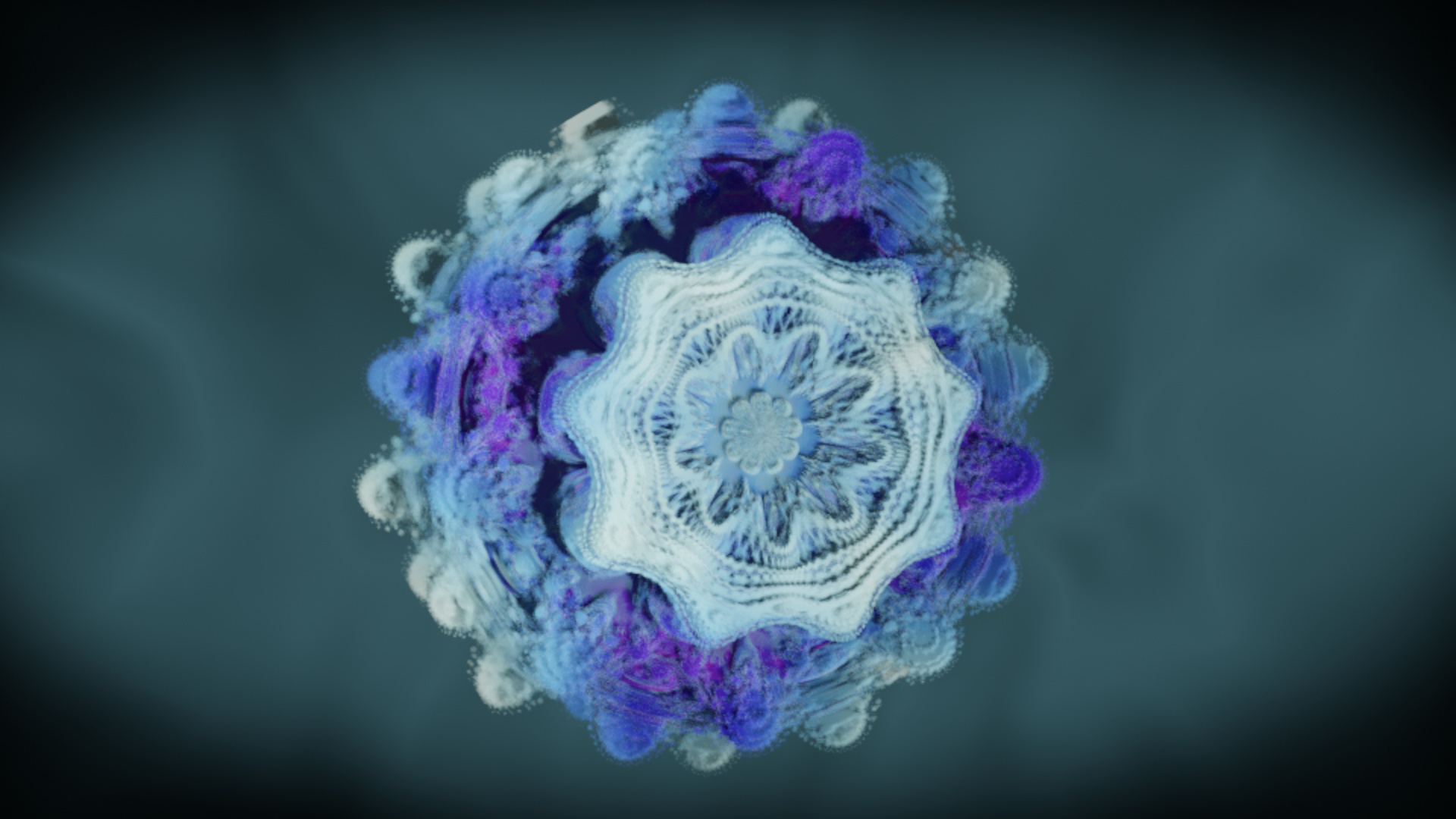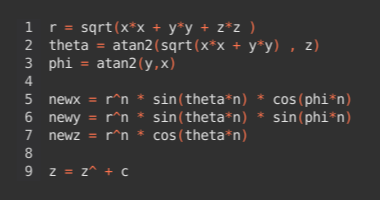# Mandelbulb

27 Oct. 2020

This is a fractal like the Mandelbrot, but in three dimensions not just in two. Fractals are a visual representation of complex mathematical formulae. In this case the formula is the following:Software used:
Blender

Rendered with:
Eevee